# 氣泡排序（Bubble Sort）、插入排序（Insertion Sort）、選擇排序（Selection Sort）

## 氣泡排序（Bubble Sort）

### 圖示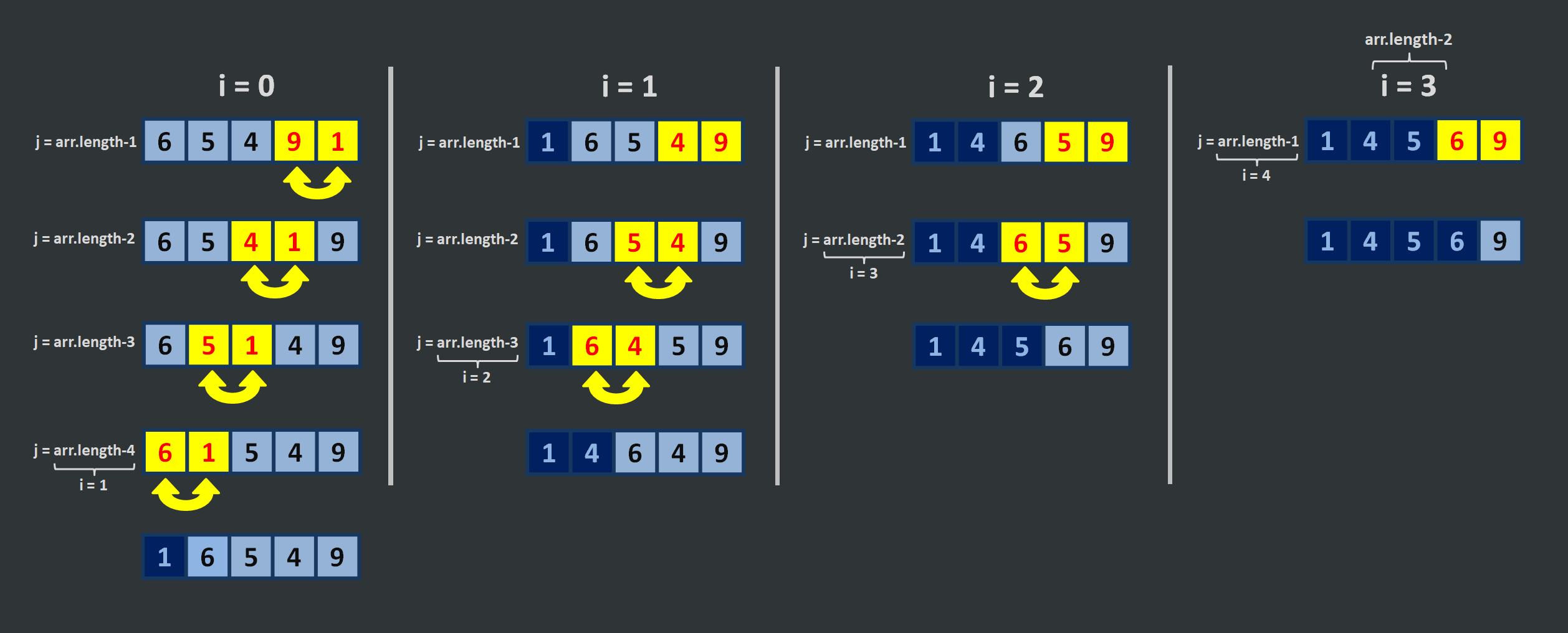### 偽代碼&說明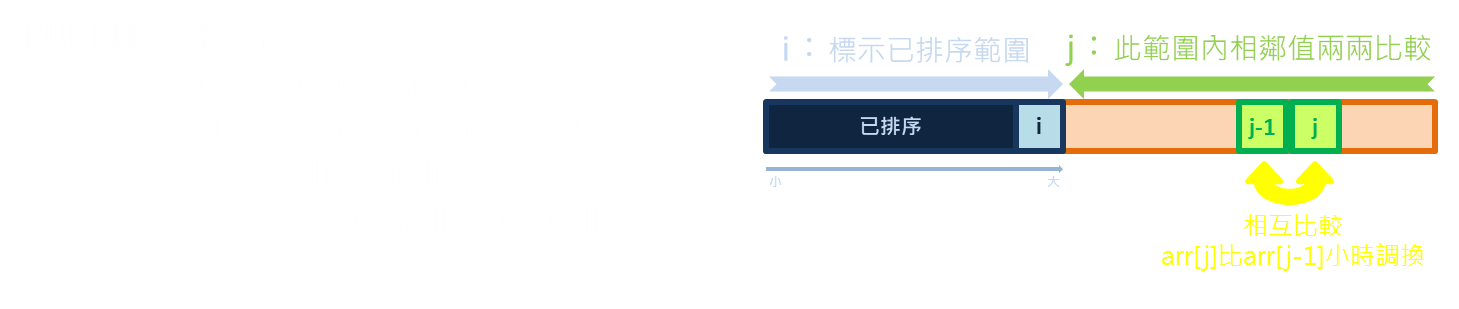### 程式碼範例（JavaScript）

``````function bubbleSort(arr){
for(let i=0; i<=arr.length-2; i++){               //用指標i標示已經排序的範圍
for(let j=arr.length-1; j>=(i+1); j--){       //指標j從尾往前走過所有未排序處
if(arr[j-1]>arr[j]){                      //指標j對應的值與相鄰的值兩兩比較，若與目標順序不同則互相調換位置
let temp = arr[j-1];
arr[j-1] = arr[j];
arr[j] = temp;
}
}
}
return arr;
}
``````

### 時間複雜度

1. 最差情況：O(n²)，若原陣列排序正好與目標順序完全相反，所有的值都被調換，最末項調換 n 次、次末項調換 (n-1) 次…第 1 項調換 1 次、第 0 項調換 0 次，全部共調換 [n+(n-1)+…+1+0] = [(n+0)·(n+1)]/2 = (n²+n)/2 次，O((n²+n)/2)=O(n²)。
2. 最佳情況：O(n)，若原陣列排序正好與目標順序完全相同，即 i 從第 0 項走到次末項，內層 j 的項目不需要執行，因此一共只有 (n-1) 個運算步驟，時間複雜度為 O(n)。
3. 平均情況：O(n²)，因使用巢狀迴圈，因此多數情況下會有 n‧n 個運算步驟。

### 空間複雜度

O(1)，在排序過程中，資料只在原陣列當中比較、調換，需要的儲存空間固定，不需額外空間暫存資料。

## 插入排序（Insertion Sort）

### 圖示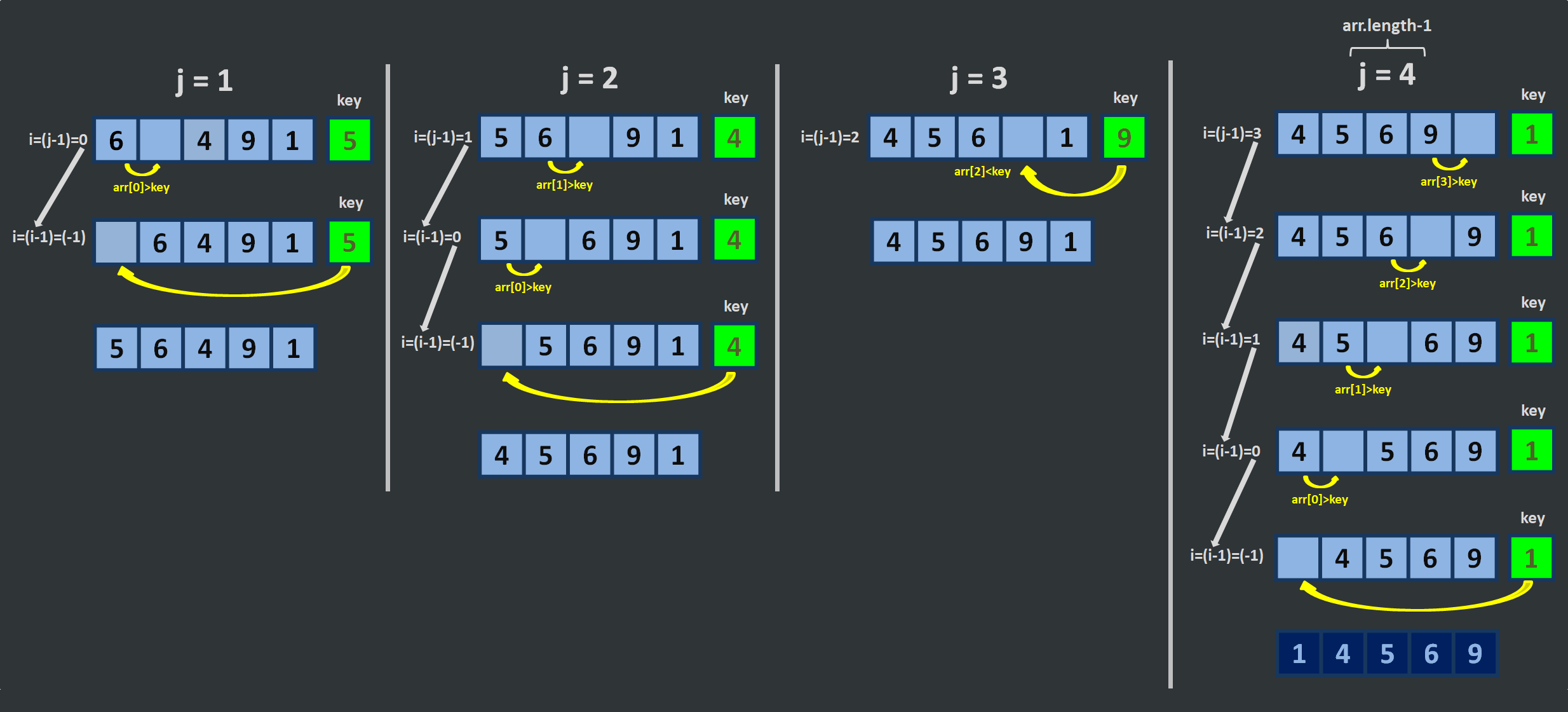### 偽代碼&說明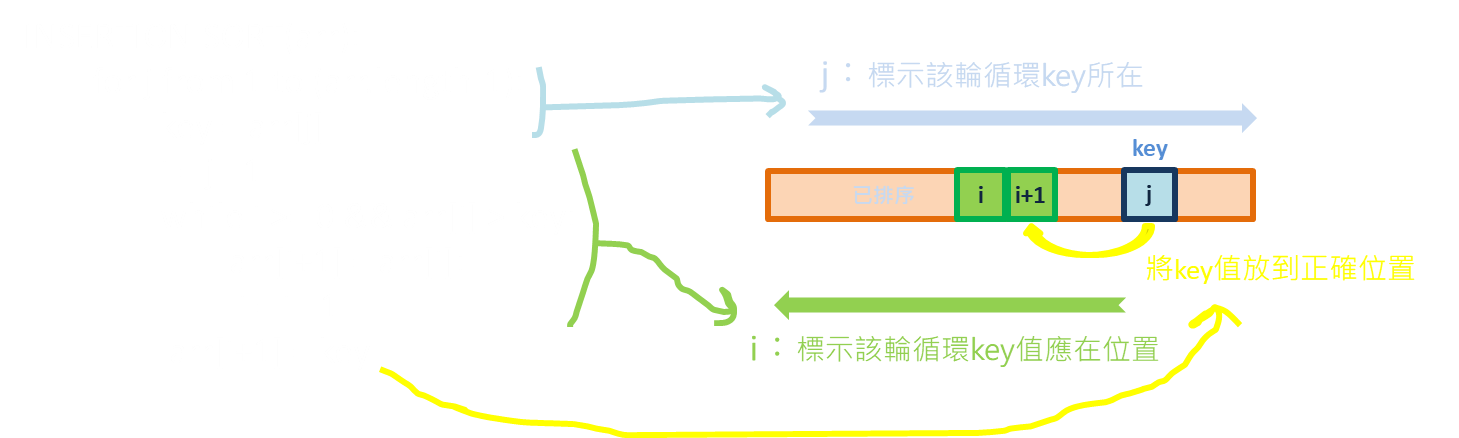### 程式碼範例（JavaScript）

``````function insertionSort(arr){
for(let j=1; j<=arr.length-1; j++){     //從第1項開始設為key，逐一跟其他資料比較
let key = arr[j];
let i = j-1;                        //連同下方while迴圈，找到該輪循環key應在位置
while(i>=0 && arr[i] > key){
arr[i+1] = arr[i];
i--;
}
arr[i+1] = key;                     //把key放到正確位置上
}
return arr;
}
``````

### 時間複雜度

1. 最差情況：O(n²)，若原陣列排序正好與目標順序完全相反，所有的值都被調換，第 0 項調換 1 次、第 1 項調換 2 次…次末項調換 (n-1) 次、最末項調換 n 次，全部共調換 [1+2...+(n-1)+n] = [(1+n)‧n]/2 = (n+n²)/2 次，O((n+n²)/2)=O(n²)。
2. 最佳情況：O(n)，若原陣列排序正好與目標順序完全相同，即 j 從第 1 項走到最末項，內層迴圈比較 i 與「key」不需執行，因此一共只有 (n-1) 個運算步驟，時間複雜度為 O(n)。
3. 平均情況：O(n²)，因使用巢狀迴圈，因此多數情況下會有 n‧n 個運算步驟。

### 空間複雜度

O(1)，在排序過程中，資料只在原陣列當中比較、賦值，需要的儲存空間固定，不需額外空間暫存資料。

## 選擇排序（Selection Sort）

### 圖示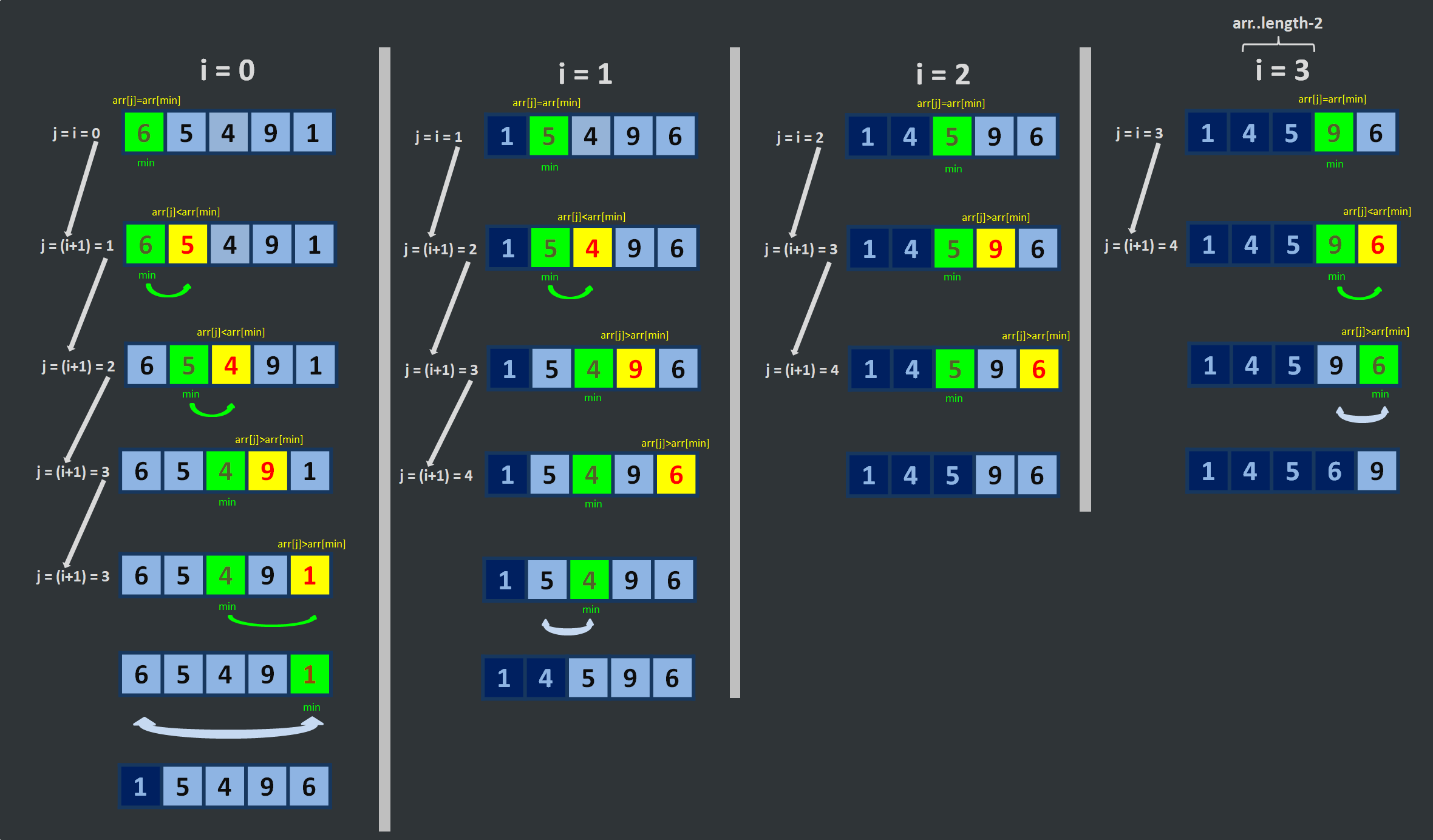### 偽代碼&說明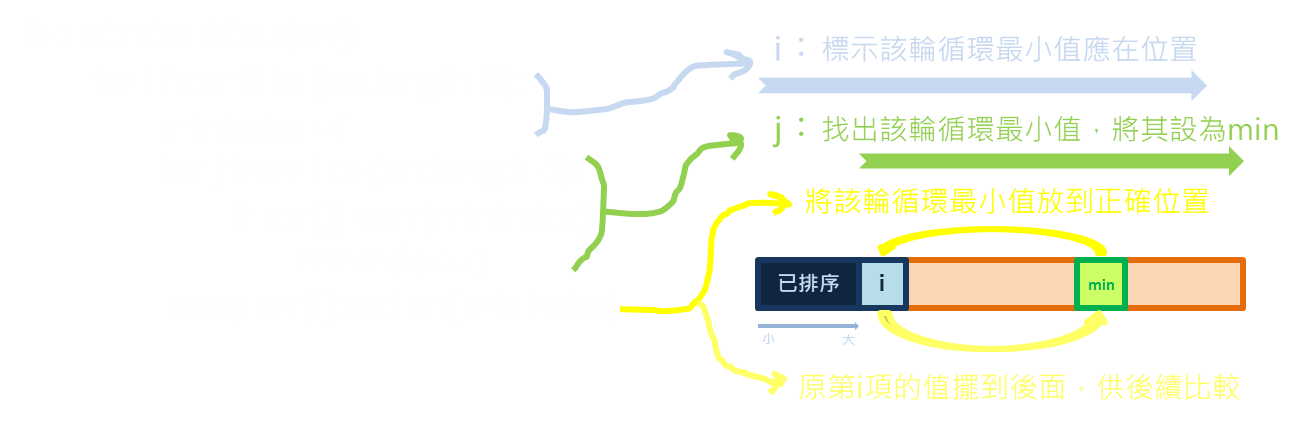### 程式碼範例（JavaScript）

``````function selectionSort(arr){
for(let i=0; i<=arr.length-2; i++){       //用指標i標示該輪循環最小值應在位置
let min = i;
for(let j=i; j<=arr.length-1; j++){   //用指標j找到該輪循環最小值，並設為第min項
if(arr[j]<arr[min]){
min = j;
}
}
temp = arr[i];                        //將該輪循環最小值從第min項換到第i項；原在第i項者被換到原第min項，待後續比較
arr[i] = arr[min];
arr[min] = temp;
}
return arr;
}
``````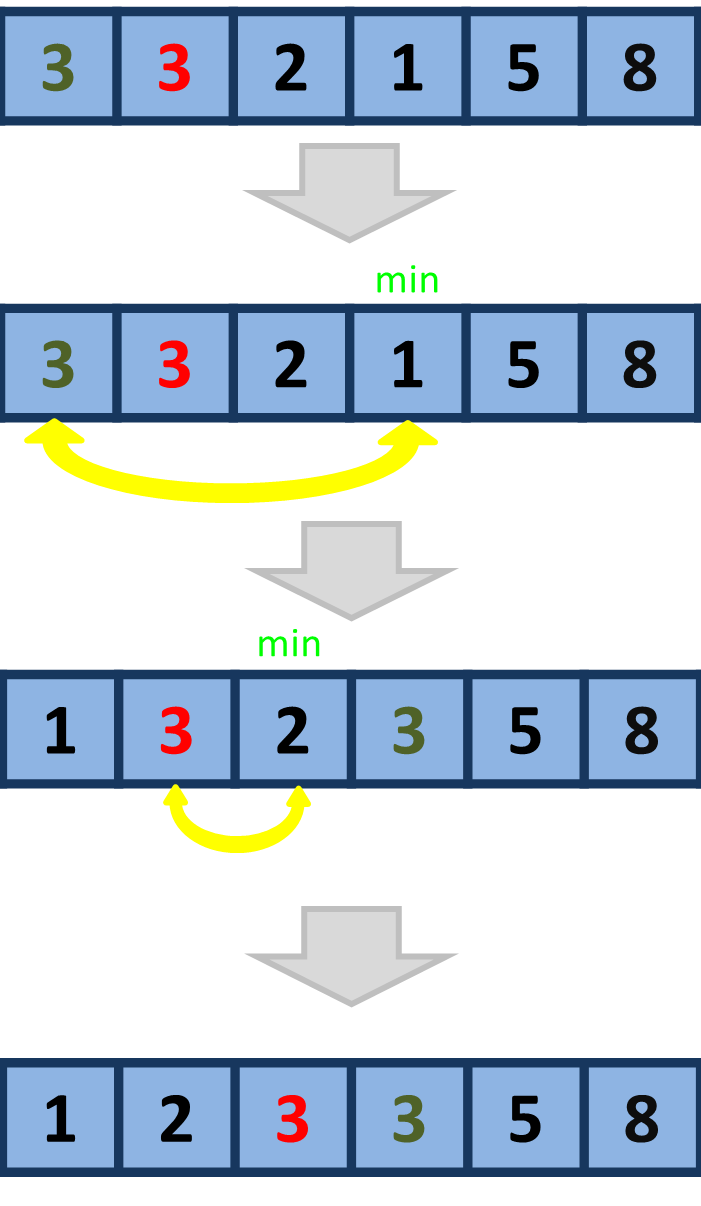### 時間複雜度

1. 最差情況：O(n²)，若原陣列排序正好與目標順序完全相反，所有的值都被調換，最末項調換 n 次、次末項調換 (n-1) 次…第 1 項調換 1 次、第 0 項調換 0 次，全部共調換 [n+(n-1)+…+1+0] = [(n+0)‧(n+1)]/2 = (n²+n)/2 次，O((n²+n)/2)=O(n²)。
2. 最佳情況：O(n)，若原陣列排序正好與目標順序完全相同，第 i 項與第 j 項依然要逐一比較，時間複雜度仍為 O(n²)。
3. 平均情況：O(n²)，因使用巢狀迴圈，不論最差或最佳情況都有 n‧n 個運算步驟，平均時間複雜度仍為 O(n²)。

### 空間複雜度

O(1)，在排序過程中，資料只在原陣列當中比較、調換，需要的儲存空間固定，不需額外空間暫存資料。

## 三種排序法複雜度比較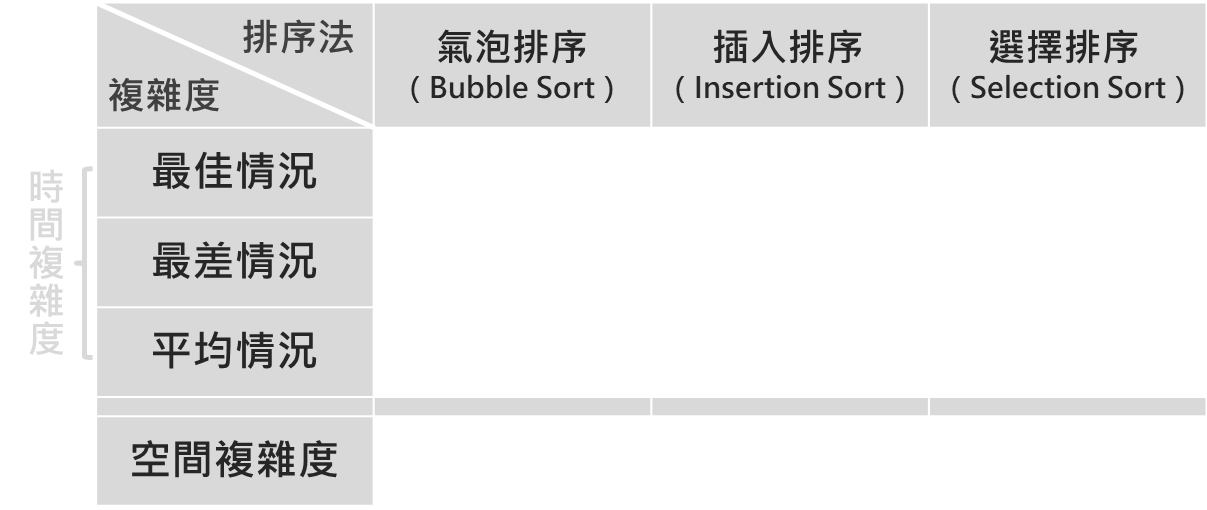#演算法 #氣泡排序 #插入排序 #選擇排序